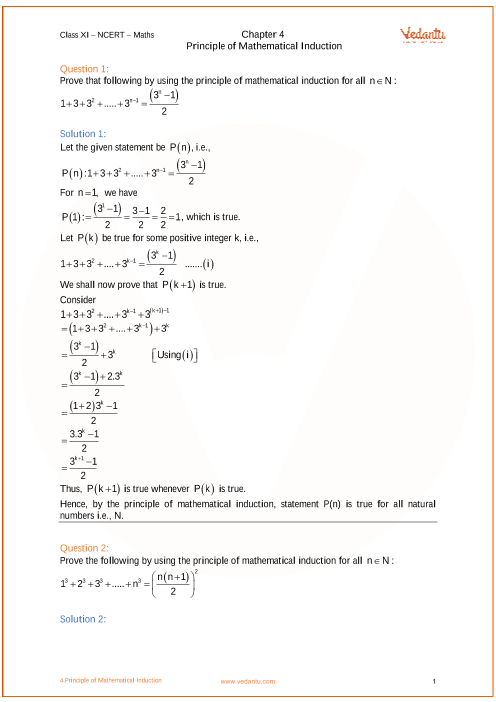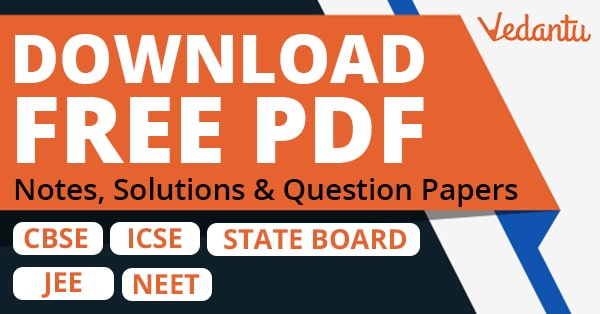# NCERT Solutions for Class 11 Maths Chapter 4 Principle of Mathematical Induction (Ex 4.1) Exercise 4.1

## NCERT Solutions for Class 11 Maths Chapter 4 Principle of Mathematical Induction (Ex 4.1) Exercise 4.1

Free PDF download of NCERT Solutions for Class 11 Maths Chapter 4 Exercise 4.1 (Ex 4.1) and all chapter exercises at one place prepared by expert teacher as per NCERT (CBSE) books guidelines. Class 11 Maths Chapter 4 Principle of Mathematical Induction Exercise 4.1 Questions with Solutions to help you to revise complete Syllabus and Score More marks. Register and get all exercise solutions in your emails.